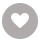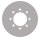# Applied Deep Learning with Keras电子书

15人正在读 | 0人评论6.8

• 读书简介
• 目录
• 累计评论(0条)
• 读书简介
• 目录
• 累计评论(0条)

Preface

Learning Objectives

Audience

Approach

Hardware Requirements

Software Requirements

Conventions

Installation and Setup

Installing the Code Bundle

Chapter 1

Introduction to Machine Learning with Keras

Introduction

Data Representation

Tables of Data

Data Preprocessing

Exercise 2: Cleaning the Data

Appropriate Representation of the Data

Exercise 3: Appropriate Representation of the Data

Life Cycle of Model Creation

Machine Learning Libraries

scikit-learn

Keras

More than Building Models

Model Training

Classifiers and Regression Models

Training and Test Datasets

Model Evaluation Metrics

Exercise 4: Creating a Simple Model

Model Tuning

Baseline Models

Exercise 5: Determining a Baseline Model

Regularization

Cross-Validation

Activity 1: Adding Regularization to the Model

Summary

Chapter 2

Machine Learning versus Deep Learning

Introduction

Hierarchical Data Representation

Linear Transformations

Scalars, Vectors, Matrices, and Tensors

Exercise 6: Perform Various Operations with Vectors, Matrices, and Tensors

Reshaping

Matrix Transposition

Exercise 7: Matrix Reshaping and Transposition

Matrix Multiplication

Exercise 8: Matrix Multiplication

Exercise 9: Tensor Multiplication

Introduction to Keras

Layer Types

Activation Functions

Model Fitting

Activity 2: Creating a Logistic Regression Model Using Keras

Summary

Chapter 3

Deep Learning with Keras

Introduction

Logistic Regression to a Deep Neural Network

Activation Functions

Forward Propagation for Making Predictions

Loss Function

Backpropagation for Computing Derivatives of Loss Function

Exercise 10: Neural Network Implementation with Keras

Activity 3: Building a Single-Layer Neural Network for Performing Binary Classification

Model Evaluation

Evaluating a Trained Model with Keras

Splitting Data into Training and Test Sets

Underfitting and Overfitting

Early Stopping

Activity 4: Diabetes Diagnosis with Neural Networks

Summary

Chapter 4

Evaluate Your Model with Cross-Validation using Keras Wrappers

Introduction

Cross-Validation

Drawbacks of Splitting a Dataset Only Once

K-Fold Cross-Validation

Leave-One-Out Cross-Validation

Comparing the K-Fold and LOO Methods

Cross-Validation for Deep Learning Models

Keras Wrapper with scikit-learn

Exercise 11: Building the Keras Wrapper with scikit-learn for a Regression Problem

Cross-Validation with scikit-learn

Cross-Validation Iterators in scikit-learn

Exercise 12: Evaluate Deep Neural Networks with Cross-Validation

Activity 5: Model Evaluation Using Cross-Validation for a Diabetes Diagnosis Classifier

Model Selection with Cross-validation

Cross-Validation for Model Evaluation versus Model Selection

Exercise 13: Write User-Defined Functions to Implement Deep Learning Models with Cross-Validation

Activity 6: Model Selection Using Cross-Validation for the Diabetes Diagnosis Classifier

Activity 7: Model Selection Using Cross-validation on the Boston House Prices Dataset

Summary

Chapter 5

Improving Model Accuracy

Introduction

Regularization

The Need for Regularization

Reducing Overfitting with Regularization

L1 and L2 Regularization

L1 and L2 Regularization Formulation

L1 and L2 Regularization Implementation in Keras

Activity 8: Weight Regularization on a Diabetes Diagnosis Classifier

Dropout Regularization

Principles of Dropout Regularization

Reducing Overfitting with Dropout

Exercise 14: Dropout Implementation in Keras

Activity 9: Dropout Regularization on Boston Housing Dataset

Other Regularization Methods

Early Stopping

Exercise 15: Implementing Early Stopping in Keras

Data Augmentation

Hyperparameter Tuning with scikit-learn

Grid Search with scikit-learn

Randomized Search with scikit-learn

Activity 10: Hyperparameter Tuning on the Diabetes Diagnosis Classifier

Summary

Chapter 6

Model Evaluation

Introduction

Accuracy

Exercise 16: Calculating Null Accuracy on a Dummy Healthcare Dataset

Imbalanced Datasets

Working with Imbalanced Datasets

Confusion Matrix

Metrics Computed from a Confusion Matrix

Exercise 17: Computing Accuracy and Null Accuracy with Healthcare Data

Activity 11: Computing the Accuracy and Null Accuracy of a Neural Network When We Change the Train/Test Split

Activity 12: Derive and Compute Metrics Based on a Confusion Matrix

Exercise 18: Calculate the ROC and AUC Curves

Summary

Chapter 7

Computer Vision with Convolutional Neural Networks

Introduction

Computer Vision

Convolutional Neural Networks

Architecture of a CNN

Input Image

Convolution Layer

Pooling Layer

Flattening

Image Augmentation

Exercise 19: Build a CNN and Identify Images of Cats and Dogs

Activity 13: Amending our Model with Multiple Layers and the Use of SoftMax

Exercise 20: Amending our model by reverting to the Sigmoid activation function

Exercise 21: Changing the Optimizer from Adam to SGD

Exercise 22: Classifying a New Image

Activity 14: Classify a New Image

Summary

Chapter 8

Transfer Learning and Pre-Trained Models

Introduction

Pre-Trained Sets and Transfer Learning

Feature Extraction

Fine-Tuning a Pre-Trained Network

The ImageNet Dataset

Some Pre-Trained Networks in Keras

Exercise 23: Identify an Image Using the VGG16 Network

Activity 15: Use the VGG16 Network to Train a Deep Learning Network to Identify Images

Exercise 24: Classification of Images That Are Not Present in the ImageNet Database.

Exercise 25: Fine-Tune the VGG16 Model

Exercise 26: Image Classification with ResNet

Activity 16: Image Classification with ResNet

Summary

Chapter 9

Sequential Modeling with Recurrent Neural Networks

Introduction

Sequential Memory and Sequential Modeling

Recurrent Neural Networks (RNNs)

Long Short-Term Memory (LSTM)

Exercise 27: Predict the Trend of Apple's Stock Price Using an LSTM with 50 Units (Neurons)

Activity 17: Predict the Trend of Microsoft's Stock Price Using an LSTM with 50 Units (Neurons)

Exercise 28: Predicting the Trend of Apple's Stock Price Using an LSTM with 100 units

Activity 18: Predicting Microsoft's Stock Price with Added Regularization

Activity 19: Predicting the Trend of Microsoft's Stock Price Using an LSTM with an Increasing Number of LSTM Neurons (100 Units)

Summary

Appendix

Chapter 1: Introduction to Machine Learning with Keras

Activity 1: Adding Regularization to the Model

Chapter 2: Machine Learning versus Deep Learning

Activity 2: Creating a Logistic Regression Model Using Keras

Chapter 3: Deep Learning with Keras

Activity 3: Building a Single-Layer Neural Network for Performing Binary Classification

Activity 4: Diabetes Diagnosis with Neural Networks

Chapter 4: Evaluate Your Model with Cross-Validation with Keras Wrappers

Activity 5: Model Evaluation Using Cross-Validation for a Diabetes Diagnosis Classifier

Activity 6: Model Selection Using Cross-Validation for the Diabetes Diagnosis Classifier

Activity 7: Model Selection Using Cross-validation on the Boston House Prices Dataset

Chapter 5: Improving Model Accuracy

Activity 8: Weight Regularization on a Diabetes Diagnosis Classifier

Activity 9: Dropout Regularization on Boston House Prices Dataset

Activity 10: Hyperparameter Tuning on the Diabetes Diagnosis Classifier

Chapter 6: Model Evaluation

Activity 11: Computing Accuracy and Null Accuracy of Neural Network When We Change the Train/Test Split

Activity 12: Derive and Compute Metrics Based on the Confusion Matrix

Chapter 7: Computer Vision with Convolutional Neural Networks

Activity 13: Amending our Model with Multiple Layers and Use of SoftMax

Activity 14: Classify a New Image

Chapter 8: Transfer Learning and Pre-trained Models

Activity 15: Use the VGG16 Network to Train a Deep Learning Network to Identify Images

Activity 16: Image Classification with ResNet

Chapter 9: Sequential Modeling with Recurrent Neural Networks

Activity 17: Predict the Trend of Microsoft’s Stock Price Using an LSTM with 50 Units (Neurons)

Activity 18: Predicting Microsoft’s stock price with added regularization

Activity 19: Predicting the Trend of Microsoft’s Stock Price Using LSTM with 100 Units

01

Michael Heydt
￥62.99
02

### Mastering OpenCV 4 with Python￥81.74

Alberto Fernández Villán
￥81.74
03

Mark J. Price
￥71.93
04

Alex Galea
￥22.99
05

Shashank Shekhar
￥54.49
06

Ajdin Imsirovic
￥53.40
07

Sergey Grinev
￥67.99
08

Gaston C. Hillar
￥62.99
09

Karol Król
￥73.02
10

### Mastering Windows PowerShell Scripting￥81.74

Chris Dent
￥81.74
•关注我们

最受欢迎的阅读产品

关注我们：

•关于我们

欢迎反馈宝贵意见给我们

客服书吧：当当云阅读问答